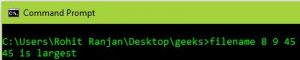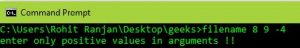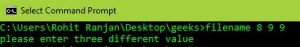# Command line arguments example in C

• Difficulty Level : Easy
• Last Updated : 25 Apr, 2022

Prerequisite: Command_line_argument. The problem is to find the largest integer among the three using command line arguments. Notes:

• Command-line arguments are given after the name of the program in the command-line shell of Operating Systems. To pass command line arguments, we typically define main() with two arguments: the first argument is the number of command-line arguments and the second is a list of command-line arguments.
`int main(int argc, char *argv[]) { /* ... */ }`
• atoi – Used to convert string numbers to integers

Examples:

```Input  : filename 8 9 45
Output : 45 is largest

Input  : filename 8 9 9
Output : Two equal number entered

Input  : filename 8 -9 9
Output : negative number entered```

When calling the program, we pass three integers along with its filename, and then the program prints out the largest of the three numbers. Approach:

1. The program “return 1” if one of the two following conditions is satisfied:
• If any two numbers are the same, print the statement “two equal numbers entered”.
• If any of the numbers is negative, print “negative number entered”.
2. Else “return 0” if three different integers are entered.

For better understanding, run this code for yourself.

## C

 `// C program for finding the largest integer``// among three numbers using command line arguments``#include ``#include ` `// Taking argument as command line``int` `main(``int` `argc, ``char` `*argv[])``{``    ``int` `a, b, c;` `    ``// Checking if number of argument is``    ``// equal to 4 or not.``    ``if` `(argc < 4 || argc > 5)``    ``{``        ``printf``("enter 4 arguments only eg.\"filename arg1 arg2 arg3!!\"");``        ``return` `0;``    ``}` `    ``// Converting string type to integer type``    ``// using function "atoi( argument)"``    ``a = ``atoi``(argv);``    ``b = ``atoi``(argv);``    ``c = ``atoi``(argv);` `    ``// Checking if all the numbers are positive of not``    ``if` `(a < 0 || b < 0 || c < 0)``    ``{``        ``printf``("enter only positive values in arguments !!");``        ``return` `1;``    ``}` `    ``// Checking if all the numbers are different or not``    ``if` `(!(a != b && b != c && a != c))``    ``{``        ``printf``("please enter three different value ");``        ``return` `1;``    ``}``    ``else``    ``{``        ``// Checking condition for "a" to be largest``        ``if` `(a > b && a > c)            ``            ``printf``("%d is largest", a);` `        ``// Checking condition for "b" to be largest   ``        ``else` `if` `(b > c && b > a)``            ``printf` `("%d is largest", b);` `        ``// Checking condition for "c" to be largest..``        ``else` `if` `(c > a && c > b)``            ``printf``("%d is largest ",c);``    ``}``    ``return` `0;``}`

Output :My Personal Notes arrow_drop_up